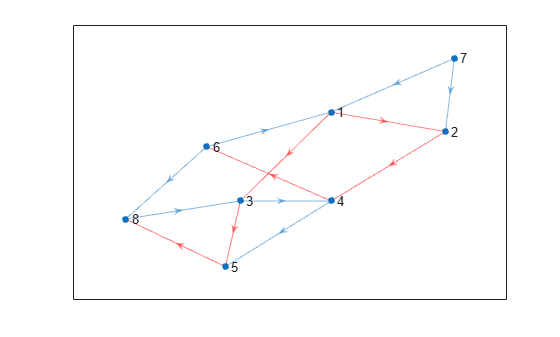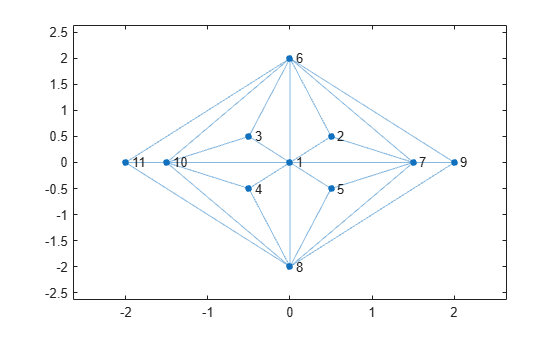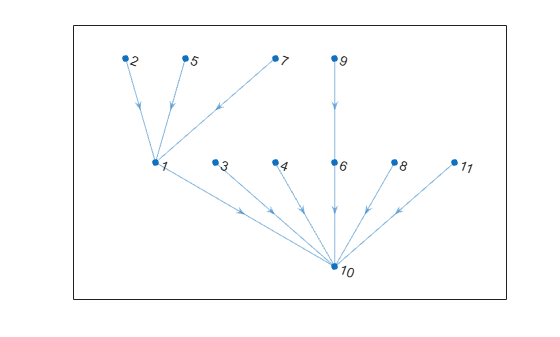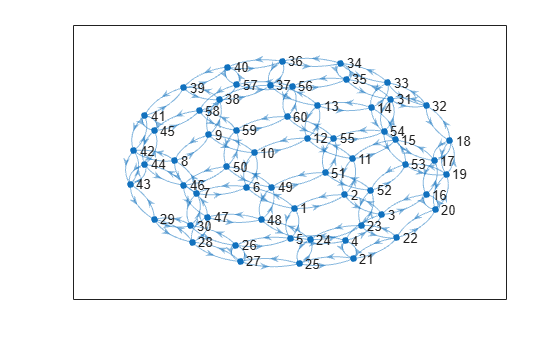# shortestpathtree

## 语法

``TR = shortestpathtree(G,s)``
``TR = shortestpathtree(G,s,t)``
``TR = shortestpathtree(___,Name,Value)``
``[TR,D] = shortestpathtree(___)``
``[TR,D,E] = shortestpathtree(___)``

## 说明

``TR = shortestpathtree(G,s)` 返回有向图 `TR`，其中包含从源节点 `s` 到图中所有其他节点的最短路径树。如果图进行了加权（即 `G.Edges` 包含变量 `Weight`），则这些权重用作沿图中各边的距离。否则，所有边距离都视为 `1`。`

``TR = shortestpathtree(G,s,t)` 计算多个源或目标节点之间的最短路径树：`s` 可以是单个源节点，`t` 可以指定多个目标节点。`s` 可以指定多个源节点，`t` 可以指定单个目标节点。`

``TR = shortestpathtree(___,Name,Value)` 使用由一个或多个名称-值对组参数指定的其他选项，这些选项使用上述语法中的任意输入参数组合。例如，`shortestpathtree(G,s,'OutputForm','vector')` 返回用于描述最短路径树的数值向量。`

``[TR,D] = shortestpathtree(___)` 还返回树中各节点之间的最短路径距离。`
``[TR,D,E] = shortestpathtree(___)` 还返回逻辑向量 `E`，指示图中的每条边是否在 `TR` 中。`

## 示例

```s = [1 1 2 3 3 4 4 6 6 7 8 7 5]; t = [2 3 4 4 5 5 6 1 8 1 3 2 8]; G = digraph(s,t)```
```G = digraph with properties: Edges: [13x1 table] Nodes: [8x0 table] ```

```TR = shortestpathtree(G,1); p = plot(G); highlight(p,TR,'EdgeColor','r')``````s = [1 1 1 1 1 1 1 2 2 7 7 7 7 9 9 3 3 1 6 4 8 10 6 8 4 5]; t = [2 3 4 5 6 8 7 6 7 5 6 8 9 6 8 6 10 10 10 10 10 11 11 11 8 8]; G = graph(s,t); x = [0 0.5 -0.5 -0.5 0.5 0 1.5 0 2 -1.5 -2]; y = [0 0.5 0.5 -0.5 -0.5 2 0 -2 0 0 0]; plot(G,'XData',x,'YData',y)``````TR = shortestpathtree(G,'all',10); plot(TR)``````G = digraph(bucky); plot(G)``````target = [1 5 13 32 44]; [TR,D] = shortestpathtree(G,23,target,'OutputForm','cell')```
```TR=5×1 cell array {[ 23 22 21 4 5 1]} {[ 23 22 21 4 5]} {[23 22 20 16 17 15 14 13]} {[ 23 22 20 19 18 32]} {[ 23 24 48 47 46 44]} ```
```D = 1×5 5 4 7 5 5 ```

`tree{j}` 是从节点 23 到节点 `target(j)` 的最短路径，长度为 `D(j)`

`path = TR{2}`
```path = 1×5 23 22 21 4 5 ```
`path_length = D(2)`
```path_length = 4 ```

## 输入参数

• 在单独使用时，`s` 必须指定单一源节点。

• `t` 一起使用时，`s``t` 输入必须满足以下条件：

• `s` 可以是单个源节点，`t` 可以指定多个目标节点。

• `s` 可以指定多个源节点，`t` 可以指定单个目标节点。

`s` 不得指定节点名称 `'all'`，因为此节点名称与选项名称冲突。对于这种情况，请改用 `findnode` 传入节点索引。

`s``t` 输入必须满足以下条件：

• `s` 可以是单个源节点，`t` 可以指定多个目标节点。

• `s` 可以指定多个源节点，`t` 可以指定单个目标节点。

`t` 不得指定名为 `'all'``'Method'``'OutputForm'` 的节点，因为这些节点名称与选项名称冲突。对于这些情况，请改用 `findnode` 传入节点索引。

### 名称-值参数

`'tree'`（默认值）

`TR` 是表示最短路径树的有向图。如果指定，则第三个输出 `E` 是一个逻辑向量，指示每条边是否在 `TR` 中。

`'cell'`

`TR` 是一个元胞数组，`TR{k}` 包含从 `s``t(k)` 或从 `s(k)``t` 的路径。如果节点之间无路径，则 `TR{k}` 为空。

`'vector'`

`TR` 是用于描述树的向量：

• 如果 `s` 只包含一个源节点，则 `TR(k)` 是从 `s``k` 的路径上的节点 `k` 之前的节点的 ID。按照惯例，`TR(s) = 0`

• 如果 `s` 包含多个源节点，则 `TR(k)` 是从 `k``k` 的路径上的节点 `t` 之后的节点的 ID。按照惯例，`TR(t) = 0`

`'auto'`（默认值）

`'auto'` 选项会自动选择算法：

• `'unweighted'` 用于没有边权重的 `graph``digraph` 输入。

• `'positive'` 用于具有边权重的所有 `graph` 输入，并要求权重为非负数。此选项还用于具有非负边权重的 `digraph` 输入。

• `'mixed'` 用于其边权重包含某些负值的 `digraph` 输入。图不能包含负循环。

`'unweighted'`

`'positive'`

Dijkstra 算法，要求所有边权重均为非负数。

`'mixed'`（仅适用于 `digraph`

`'acyclic'`（仅适用于 `digraph`

## 输出参数

• 如果不指定 `'OutputForm'` 或者指定了 `'tree'` 的值，则 `E` 是一个逻辑向量，指示图中的每条边是否在有向图 `TR` 中。此输出与 `highlight``'Edges'` 名称-值对组兼容，例如：`highlight(p,'Edges',E)`

• 如果 `'OutputForm'``'cell'`，则 `E` 是一个元胞数组，其中包含 `TR` 中对应路径上的边。

• 如果 `'OutputForm'``'vector'`，则 `E` 是一个向量，给出最短路径树中将每个节点连接到父节点的边的索引。

## 提示

• `shortestpath``shortestpathtree``distances` 函数不支持具有负边权重的无向图，或更通俗地说，不支持包含负循环的任何图，原因如下：

• 负循环是从节点出发回到自身的路径，路径上的边权重之和为负值。如果两个节点之间的路径上具有负循环，则这两个节点之间不存在最短路径，因为始终可以通过遍历负循环找到更短路径。

• 无向图中的单个负边权重会创建一个负循环。